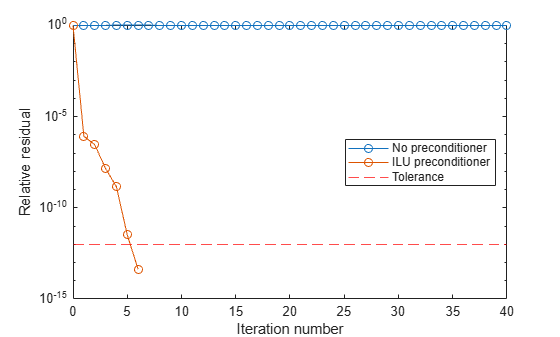Documentation

# tfqmr

Transpose-free quasi-minimal residual method

## Syntax

```x = tfqmr(A,b) x = tfqmr(afun,b) x = tfqmr(a,b,tol) x = tfqmr(a,b,tol,maxit) x = tfqmr(a,b,tol,maxit,m) x = tfqmr(a,b,tol,maxit,m1,m2,x0) [x,flag] = tfqmr(A,B,...) [x,flag,relres] = tfqmr(A,b,...) [x,flag,relres,y]y(A,b,...) [x,flag,relres,iter,resvec] = tfqmr(A,b,...) ```

## Description

`x = tfqmr(A,b)` attempts to solve the system of linear equations `A*x=b` for `x`. The `n`-by-`n` coefficient matrix `A` must be square and the right-hand side column vector `b` must have length `n`.

`x = tfqmr(afun,b)` accepts a function handle, `afun`, instead of the matrix `A`. The function, `afun(x)`, accepts a vector input `x` and returns the matrix-vector product `A*x`. In all of the following syntaxes, you can replace `A` by `afun`. Parameterizing Functions explains how to provide additional parameters to the function `afun`.

`x = tfqmr(a,b,tol)` specifies the tolerance of the method. If `tol` is `[]` then `tfqmr` uses the default, 1e-6.

`x = tfqmr(a,b,tol,maxit)` specifies the maximum number of iterations. If `maxit` is `[]` then `tfqmr` uses the default, `min(N,20)`.

`x = tfqmr(a,b,tol,maxit,m)` and ```x = tfqmr(a,b,tol,maxit,m1,m2)``` use preconditioners `m` or `m=m1*m2` and effectively solve the system ```A*inv(M)*x = B``` for `x`. If `M` is `[]` then a preconditioner is not applied. `M` may be a function handle `mfun` such that `mfun(x)` returns `m\x`.

`x = tfqmr(a,b,tol,maxit,m1,m2,x0)` specifies the initial guess. If `x0` is `[]` then `tfqmr` uses the default, an all zero vector.

`[x,flag] = tfqmr(A,B,...)` also returns a convergence flag:

Flag

Convergence

`0`

`tfqmr` converged to the desired tolerance `tol` within `maxit` iterations.

`1`

`tfqmr` iterated `maxit` times but did not converge.

`2`

Preconditioner `m` was ill-conditioned.

`3`

`tfqmr` stagnated. (Two consecutive iterates were the same.)

`4`

One of the scalar quantities calculated during `tfqmr` became too small or too large to continue computing.

`[x,flag,relres] = tfqmr(A,b,...)` also returns the relative residual `norm(b-A*x)/norm(b)`. If `flag` is 0, then `relres <= tol`.

`[x,flag,relres,y]y(A,b,...)` also returns the iteration number at which `x` was computed: ```0 <= iter <= maxit```.

`[x,flag,relres,iter,resvec] = tfqmr(A,b,...)` also returns a vector of the residual norms at each iteration, including `norm(b-A*x0)`.

## Examples

### Using tfqmr with Matrix or Function Handle Input

This example shows how to use `tfqmr` with a matrix input and with a function input.

```n = 100; on = ones(n,1); A = spdiags([-2*on 4*on -on],-1:1,n,n); b = sum(A,2); tol = 1e-8; maxit = 15; M1 = spdiags([on/(-2) on],-1:0,n,n); M2 = spdiags([4*on -on],0:1,n,n); x = tfqmr(A,b,tol,maxit,M1,M2,[]);```

You can also use a matrix-vector product function as input:

```function y = afun(x,n) y = 4 * x; y(2:n) = y(2:n) - 2 * x(1:n-1); y(1:n-1) = y(1:n-1) - x(2:n); x1 = tfqmr(@(x)afun(x,n),b,tol,maxit,M1,M2);```

If `applyOp` is a function suitable for use with `qmr`, it may be used with `tfqmr` by wrapping it in an anonymous function:

`x1 = tfqmr(@(x)applyOp(x,'notransp'),b,tol,maxit,M1,M2);`

### Using tfqmr with a Preconditioner

This example demonstrates the use of a preconditioner.

Load `A = west0479`, a real 479-by-479 nonsymmetric sparse matrix.

```load west0479; A = west0479;```

Define `b` so that the true solution is a vector of all ones.

`b = full(sum(A,2));`

Set the tolerance and maximum number of iterations.

```tol = 1e-12; maxit = 20;```

Use `tfqmr` to find a solution at the requested tolerance and number of iterations.

`[x0,fl0,rr0,it0,rv0] = tfqmr(A,b,tol,maxit);`

`fl0` is 1 because `tfqmr` does not converge to the requested tolerance `1e-12` within the requested 20 iterations. The seventeenth iterate is the best approximate solution and is the one returned as indicated by `it0 = 17`. MATLAB® stores the residual history in `rv0`.

Plot the behavior of `tfqmr`.

```semilogy(0:maxit,rv0(1:maxit+1)/norm(b),'-o'); xlabel('Iteration number'); ylabel('Relative residual');```Note that like `bicgstab`, `tfqmr` keeps track of half iterations. The plot shows that the solution does not converge. You can use a preconditioner to improve the outcome.

Create the preconditioner with `ilu`, since the matrix `A` is nonsymmetric.

```[L,U] = ilu(A,struct('type','ilutp','droptol',1e-5)); ```
```Error using ilu There is a pivot equal to zero. Consider decreasing the drop tolerance or consider using the 'udiag' option. ```

MATLAB cannot construct the incomplete LU as it would result in a singular factor, which is useless as a preconditioner.

You can try again with a reduced drop tolerance, as indicated by the error message.

```[L,U] = ilu(A,struct('type','ilutp','droptol',1e-6)); [x1,fl1,rr1,it1,rv1] = tfqmr(A,b,tol,maxit,L,U);```

`fl1` is 0 because `tfqmr` drives the relative residual to `4.1410e-014` (the value of `rr1`). The relative residual is less than the prescribed tolerance of `1e-12` at the sixth iteration (the value of `it1`) when preconditioned by the incomplete LU factorization with a drop tolerance of `1e-6`. The output `rv1(1)` is `norm(b)`, and the output `rv1(7)` is `norm(b-A*x2)`.

You can follow the progress of `tfqmr` by plotting the relative residuals at each iteration starting from the initial estimate (iterate number 0).

```semilogy(0:0.5:it1,rv1/norm(b),'-o'); xlabel('Iteration number'); ylabel('Relative residual');```